# Division

1
One number is 1
2
One number is 2
3
One number is 3
4
One number is 4
5
One number is 5
6
One number is 6
7
One number is 7
8
One number is 8
9
One number is 9
10
One number is 10
11
One number is 11
12
One number is 12
13
One number is 13
14
One number is 14
15
One number is 15
16
One number is 16
17
One number is 17
18
One number is 18
19
One number is 19
20
One number is 20
21
One number is 21-25
22
One number is 26-30
23
One number is 31-35
24
One number is 36-40
25
One number is 41-45
26
One number is 46-50
27
One number is 51-55
28
One number is 56-60
29
One number is 61-65
30
One number is 66-70
31
One number is 71-75
32
One number is 76-80
33
One number is 81-85
34
One number is 86-90
35
One number is 91-100
Difficult
Do you want to play locked game level?

## Recent activities in this game

Someone got all questions correct at level 5. One number is 5 .
1 hour ago after 6 seconds of playing.
Someone got all questions correct at level 4. One number is 4 .
1 hour ago after 9 seconds of playing.
Someone got all questions correct at level 3. One number is 3 .
1 hour ago after 14 seconds of playing.
Someone got all questions correct at level 2. One number is 2 .
1 hour ago after 8 seconds of playing.
Someone got all questions correct at level 1. One number is 1 .
1 hour ago after 9 seconds of playing.
Someone had a problem at level 1. One number is 1 .
15 hours ago after 30 seconds of playing.
A student got all questions correct at level 4. One number is 4 .
2023-12-08 23:04 after 21 seconds of playing.
A student got all questions correct at level 4. One number is 4 .
2023-12-08 23:03 after 9 seconds of playing.
A student got all questions correct at level 4. One number is 4 .
2023-12-08 23:03 after 10 seconds of playing.
A student got all questions correct at level 4. One number is 4 .
2023-12-08 22:59 after 13 seconds of playing.

Can you divide with integer numerators and denominators? Division skill practice in a fun math game.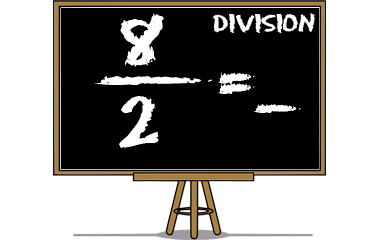## Game instructions for division

Choose a number from the buttons above. This will give you up to ten fractions where you have to count division and find the quotient. Can you solve all questions, you get one knowledge point and the numbers button will be marked green.

### Division in the language of mathematics

Division is one of the four basic rules of arithmetic and you can either talk about dividing two numbers or finding the quotient between two numbers.

Numerator divided by the denominator results in a quotient:
 6 / 2 = 3 numerator / denominator = quotient

### Calculation of knowledge points

Each completed game level gives 1 knowledge point in Division. The maximum number of points (35 knowledge points) is achieved when you pass all 35 levels. You'll get a bronze medal when you complete a level 2 times and a silver medal after 5 completed rounds. A gold medal will be received after 10 completed rounds. In Division, the maximum number of collectable gold medals is 35. You only earn knowledge points for levels that has not been cleared before. A cleared level has a green background above. Even when you have completed a level, you can continue practicing at that level, but it gives you no more knowledge points.

### Similar gamesMath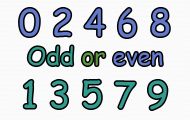Math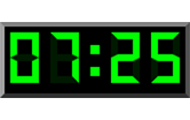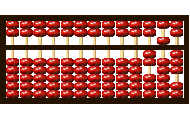Math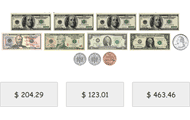Math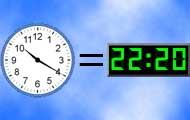Math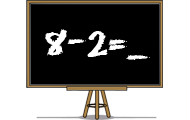Math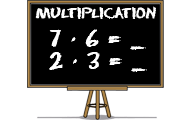Math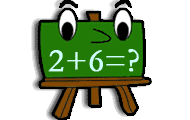Math

Subject
Math
Target group
Practices
Numbers Division Mental calculation
Game creator
Updated
2019-10-02
Published online
2011-11-11
Unique id
10
Saved by
5-10 users
Operating system
Browser
Price
Free of charge

4
Total 175
5
120
4
10
3
8
2
4
1
33

### Report

If you find a spelling mistake or something else that should be corrected, feel free to contact the game creator or us at Helpful Games.

Write a comment below to contact the game creator or

H
Write a comment and tell other visitors!
Most played
1.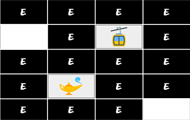Brain training
2.Brain training
3.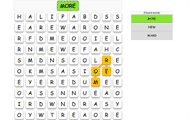English
4.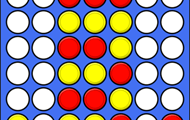Brain training
5.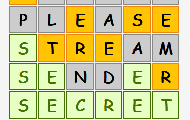English

More plus membership benefits